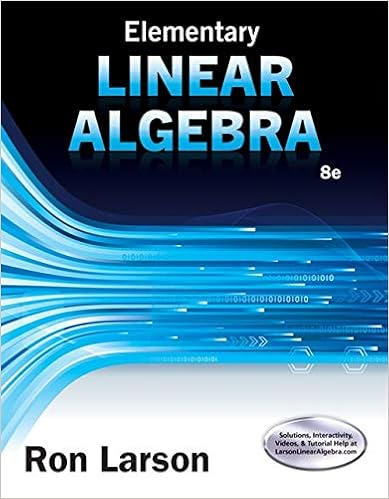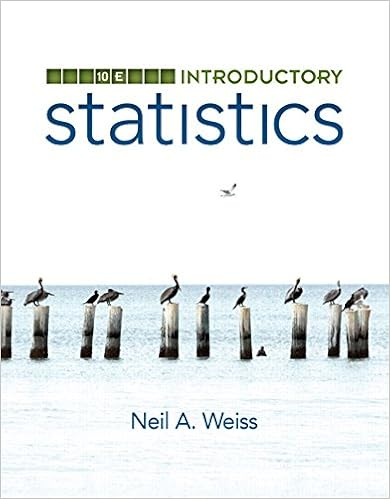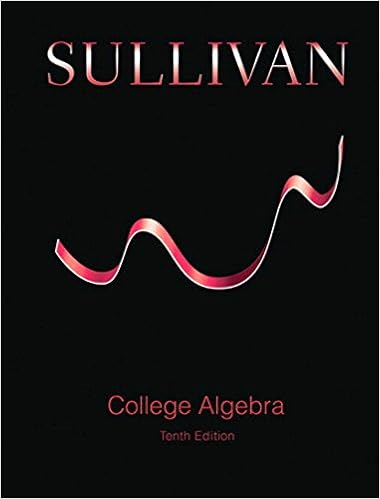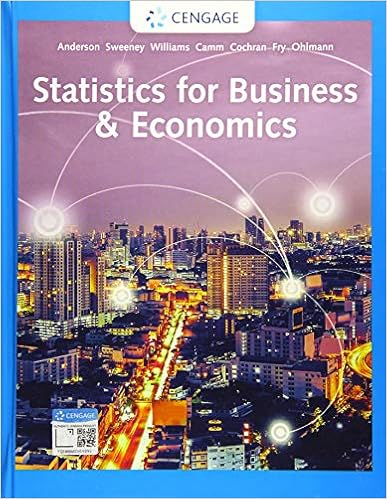# hw6 - Math 307: Assignment 6 Please look at the Course News...

• Homework Help
• 3
• 100% (2) 2 out of 2 people found this document helpful

This preview shows page 1 - 2 out of 3 pages.

The preview shows page 1 - 2 out of 3 pages.
Math 307: Assignment 6Please look at the Course News to see which of the follwing problems need to be solved.Definitely,solving all of the problems would be helpful for the midterm exam but it is not mandatory.1.Compute the 1,2 and infinity norms for the following vectors. Is it always the same normthat is biggest? (The 2-norm is the standard Euclidean norm)
2.Find four vectors in two dimensions whose 1, 2 and infinity norms the same.3.Suppose you were doing a problem each entryviin a vector[v1,v2,...,vn]is positive andrepresents the yearly production of one ofnfactories.Which of the three norms weintroduced have natural interpretations in this context.4.Draw a picture of the “unit circle” for the 1,2, and infinty norms in two dimensions. By“unit circle” we mean the set of all vectors whose norm is equal to one.5.Recall that the Euclidean distance between two vectors v and w isbardblvwbardblwhere we usethe standard norm. If we use the1-norm or-norm in this formula, we obtain differentdistance functions.Consider vectors whose entries are either0or1(like[0,1,1,0,1]).Describe in words the meaning of the1-distance and the-distance between two suchvectors.6.Use MATLAB/Octave to compute both sides of the triangle inequalitybardblx+ybardbl ≤ bardblxbardbl+bardblybardblfor some random vectors x and y and for each of the norms (1,2and) that we defined.Verify that for these examples the inequality is true.7.Thep-norm of a vector v= [v1,v2,...,vn]Tfor1p≤ ∞is defined to bebardblvbardblp=parenleftBiggnsummationdisplayi=1|vi|p

Course Hero member to access this document

##### We have textbook solutions for you!The document you are viewing contains questions related to this textbook.
Chapter 7 / Exercise 33
Elementary Linear Algebra
LarsonExpert VerifiedBrowse all Textbook Solutions

Course Hero member to access this document

End of preview. Want to read all 3 pages?

Course Hero member to access this document

Term
Fall
Professor
RICHARDFROESE
Tags
Math, Euclidean space
##### We have textbook solutions for you!
The document you are viewing contains questions related to this textbook.The document you are viewing contains questions related to this textbook.
Chapter 7 / Exercise 33
Elementary Linear Algebra
LarsonExpert Verified
•••